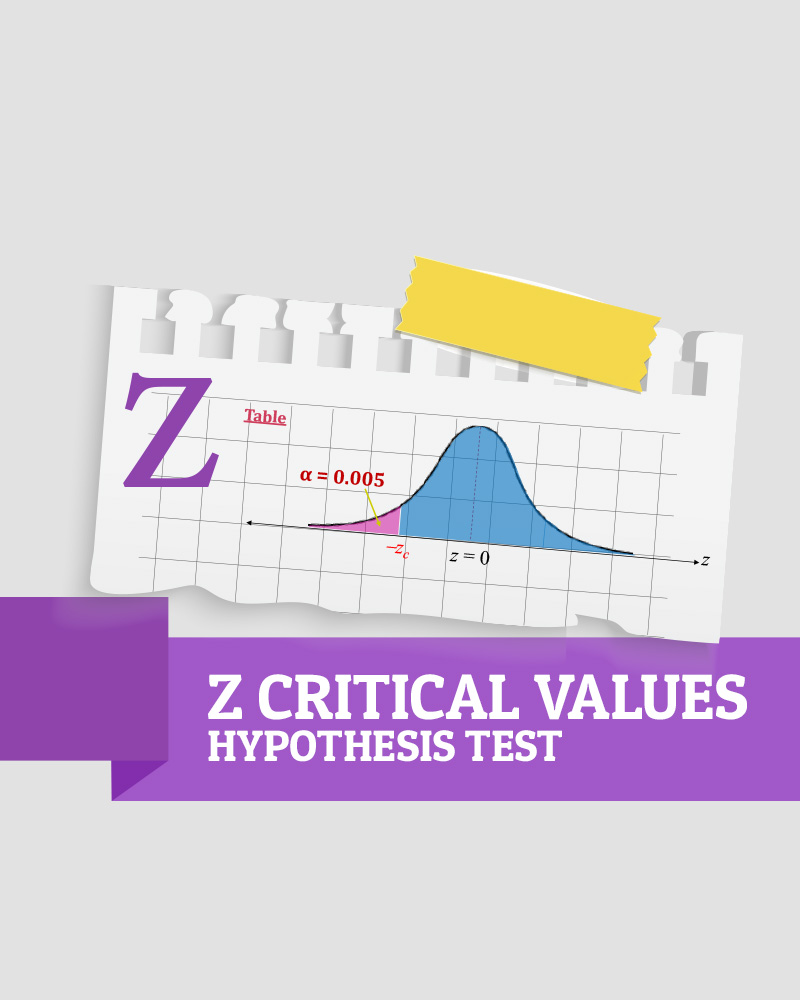# Finding z critical values for Hypothesis test

Description.

• In summary, in order to find z-critical values, we need to find the left cumulative area to look up the z-table.
• For left-tailed tests, since we have the left area as the significance level for the test, we use that α to find the z critical value
• However, for right-tailed tests, we need to subtract the significance level from 1 to get the left cumulative area.
• Lastly, for two-tailed tests, simply divide the significance level by 2 to get the left area. Once you get the negative critical value, then make it positive to have two critical values.